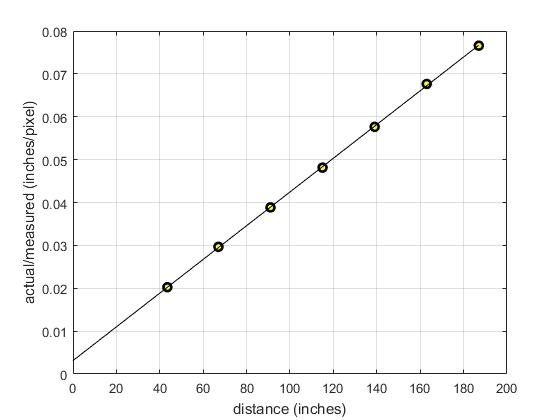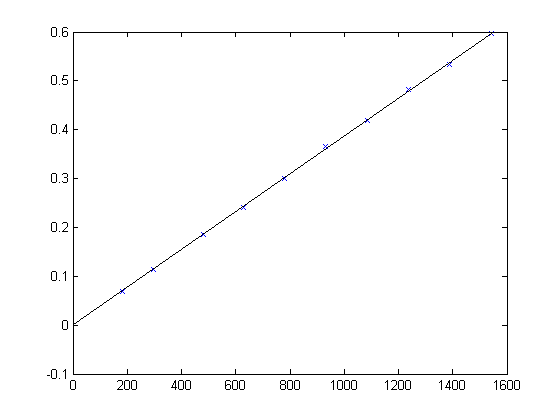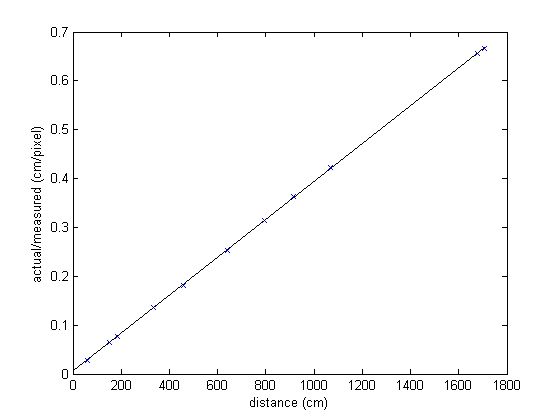# Camera Calibration - Focal Length

MATLAB source: efl_calc.zip.

Focal length can be determined from a series of images of a target board at varying distances between the camera and the target. The camera used here is a Fugifilm Finepix 6900 with zoom lens set at its power-on value.

Our analysis consists of the following steps.

1. List the image filenames so that we don't have to type them manually. We use the MATLAB code listing.m for this purpose. This generates a set of data in `filelist.mat`

2. Pick a set of images and display them, as shown in img_array.m.

3. Use the mouse to locate the corners of our target. The MATLAB function `ginput` is called to solicit user input. See the MATLAB code img_meas.m. The results are stored in `imgdata.mat`. The results are displayed graphically with img_array_data.m.

4. Use the measured actual size of the target wo, the measurement of distance z for each image, and the imaged target sizes wi. Plot wo/wi vs. z and determine the best-fitting line (using the MATLAB function `polyfit`). Determine the focal length from the slope of this line. See the results in efl_calc.m. The plot is also shown belowThe desired parameter value is f = 2542.3 pixels

## Previous Measurements

Distances were measured in cm. For comparison, 200 inches (above) corresponds to about 500 cm

1. 2012_0327. f = 2581.13 pixels.2. 2012_0328. f = 2576.21 pixels.Maintained by John Loomis, last updated 2 June 2012